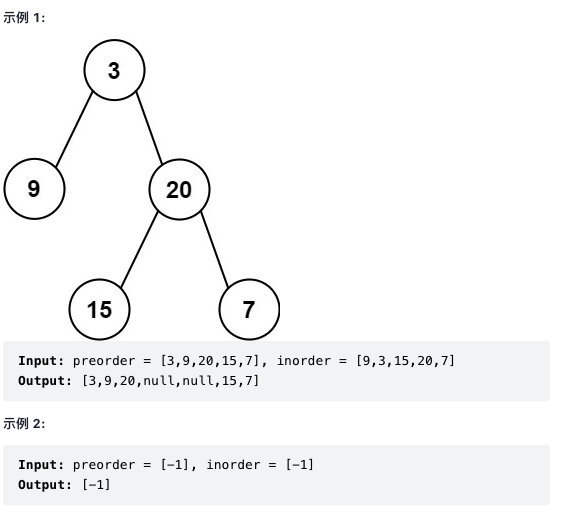# 手撸二叉树之从前序与中序遍历序列构造二叉树HelloWorld杰少Hello, 大家好，今天是我参加 9 月更文的第 12 天，今天给大家带来的关于二叉树相关的算法题是从前序与中序遍历构建二叉树，正文如下：

## 题目## 代码实现

/** * Definition for a binary tree node. * public class TreeNode { *     int val; *     TreeNode left; *     TreeNode right; *     TreeNode() {} *     TreeNode(int val) { this.val = val; } *     TreeNode(int val, TreeNode left, TreeNode right) { *         this.val = val; *         this.left = left; *         this.right = right; *     } * } */class Solution {    public HashMap<Integer, Integer> map = new HashMap();    int[] preOrder;    public TreeNode buildTree(int[] preorder, int[] inorder) {        for (int i = 0; i < inorder.length; i++) {            map.put(inorder[i], i);        }        this.preOrder = preorder;        return helper(0, inorder.length - 1, 0, preOrder.length - 1);    }    public TreeNode helper(int inStart, int inEnd, int preStart, int preEnd) {        if (inStart > inEnd || preStart > preEnd) return null;        int root = preOrder[preStart];         int pos = map.get(root);        TreeNode node = new TreeNode(root);        node.left = helper(inStart, pos - 1, preStart + 1, preStart + pos - inStart);        node.right = helper(pos + 1, inEnd, preStart + pos - inStart + 1, preEnd);        return node;    }}

## 最后

• 时间复杂度：O(n)，其中 n 是树中的节点个数。

• 空间复杂度：O(n)，除去返回的答案需要的 O(n) 空间之外，我们还需要使用 O(n) 的空间存储哈希映射，以及 O(h)（其中 h 是树的高度）的空间表示递归时栈空间。这里 h < n，所以总空间复杂度为 O(n)。## 评论